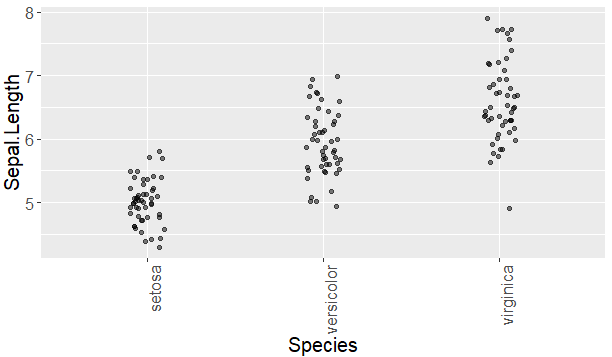2 views

I am plotting a graph with a categorical variable on the x axis and a numerical variable on the y axis.

For the x axis, given that there are many data points, the default text formatting causes the label for each tick mark to overlap with other labels. How do I (a) change the font size for my axis text and (b) change the orientation of the text so that the text is perpendicular to the axis?

by

To change the font size and direction of the axes, add the following function to your plot:

theme(text = element_text(size=15),axis.text.x = element_text(angle=90, hjust=1))

angle rotates the text, and hjust controls the horizontal justification.

For example:

data(iris)

ggplot(iris, aes(x=Species, y=Sepal.Length)) +

geom_jitter(width = 0.1, alpha = 0.5) +

theme(text = element_text(size=15),axis.text.x = element_text(angle=90, hjust=1))

Output: